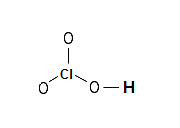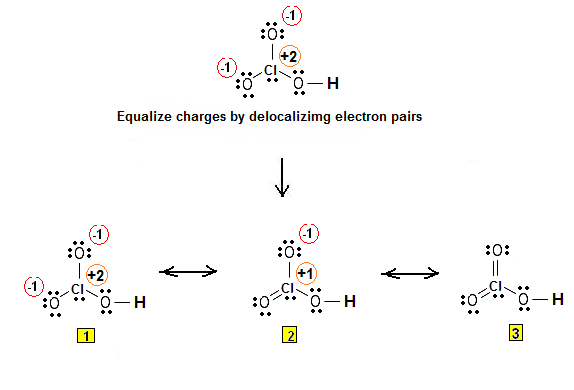Lewis Electron Dot Structures - Simple Procedure for writing Lewis Structures of Chloric Acid (HClO3) | Chemistry Net

# Lewis Electron Dot Structures - Simple Procedure for writing Lewis Structures of Chloric Acid (HClO3)

Lewis Electron Dot Structures - Simple Procedure for writing Lewis Structures of Chloric Acid (HClO3)

# Lewis Electron Dot Structure of Chloric Acid HClO3

A simple procedure for writing Lewis structures is given in a previous article entitled “Lewis Structures and the Octet Rule”. Several worked examples relevant to this procedure were given in previous posts please see the Sitemap - Table of Contents (Lewis Electron Dot Structures).

Another example  for writing Lewis structures following the above procedure is given below.

Let us consider the case of the electron dot structures of chloric acid HClO3. Chloric acid is a colorless liquid. It will accelerate the burning of combustible materials and can ignite most on contact. It is a strong oxidizing agent. It is used as a reagent in chemical analysis and to make other chemicals.

How can we construct the Lewis structure of HClO3 ?

Step 1: Connect the atoms with single bonds. Chlorine is the central atom:Step 2: Calculate the # of electrons in π bonds (multiple bonds) using  formula (1) in the article entitled “Lewis Structures and the Octet Rule”.

Where n in this case is 4 since HClO3 consists of 5 atoms but one of them is a hydrogen atom (remember n is the number of atoms in a molecule minus the hydrogen atoms).

Where V = (1 + 7 + 3 * 6 ) = 26

Therefore, P = 6n + 2 – V = 6 * 4 + 2 – 26 = 0 and therefore there are no π electrons in HClO3.

So the structure of Step 1 is the Lewis structure.

Electrons are placed around each atom so that the octet rule is obeyed. Formal charges are assigned and equalized using resonance.

Step 3 & 4: The Lewis structure for HClO3 is as follows:Relevant Posts - Relevant Videos

Lewis Structures|Octet Rule: A Simple Method to write Lewis Structures

Simple Method for writing Lewis Structures of Perchloric Acid HClO4

References

1. G.N. Lewis, J.A.C.S, 38, 762-785, (1916)
2. E. C. McGoran, J. Chem. Educ., 68, 19-23 (1991)
3. A.B.P. Lever, J. Chem. Educ., 49, 819-821, (1972)

Key Terms

resonance structures of chloric acid HClO3 chloric acid, Lewis electron structures of chloric acid, chemical formula of HClO3 chloric acid, simple procedure for drawing Lewis structures of chloric acid,